## Determinant

Determinants are mathematical objects which are very useful in the analysis and solution of systems of linear equations. As shown in Cramer's Rule, a nonhomogeneous system of linear equations has a nontrivial solution Iff the determinant of the system's Matrix is Nonzero (so that the Matrix is nonsingular). A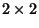determinant is defined to be(1)

Adeterminant can be expanded by Minors to obtain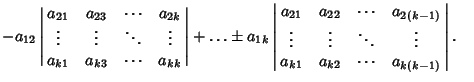(2)
A general determinant for a Matrix A has a value(3)

with no implied summation over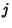and whereis the Cofactor of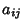defined by(4)

Here, C is the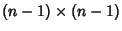Matrix formed by eliminating rowand columnfrom A, i.e., by Determinant Expansion by Minors.

Given andeterminant, the additive inverse is(5)

Determinants are also Distributive, so(6)

This means that the determinant of a Matrix Inverse can be found as follows:(7)

where I is the Identity Matrix, so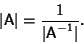(8)

Determinants are Multilinear in rows and columns, since(9)

and(10)

The determinant of the Similarity Transformation of a matrix is equal to the determinant of the original Matrix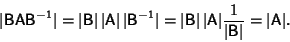(11)

The determinant of a similarity transformation minus a multiple of the unit Matrix is given by(12)

The determinant of a Matrix Transpose equals the determinant of the original Matrix,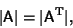(13)

and the determinant of a Complex Conjugate is equal to the Complex Conjugate of the determinant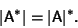(14)

Let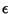be a small number. Then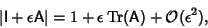(15)

whereis the Trace of A. The determinant takes on a particularly simple form for a Triangular Matrix(16)

Important properties of the determinant include the following.

1. Switching two rows or columns changes the sign.

2. Scalars can be factored out from rows and columns.

3. Multiples of rows and columns can be added together without changing the determinant's value.

4. Scalar multiplication of a row by a constant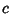multiplies the determinant by.

5. A determinant with a row or column of zeros has value 0.

6. Any determinant with two rows or columns equal has value 0.

Property 1 can be established by induction. For aMatrix, the determinant is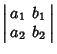(17)

For aMatrix, the determinant is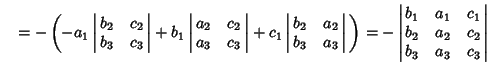(18)
Property 2 follows likewise. Forandmatrices,(19)

and(20)

Property 3 follows from the identity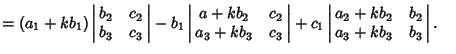(21)

Ifis anMatrix withReal Numbers, thenhas the interpretation as the oriented-dimensional Content of the Parallelepiped spanned by the column vectors, ...,in. Here, oriented'' means that, up to a change ofor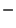Sign, the number is the-dimensional Content, but the Sign depends on the orientation'' of the column vectors involved. If they agree with the standard orientation, there is aSign; if not, there is aSign. The Parallelepiped spanned by the-D vectors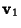through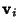is the collection of points(22)

where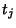is a Real Number in the Closed Interval [0,1].

There are an infinite number ofdeterminants with no 0 orentries having unity determinant. One parametric family is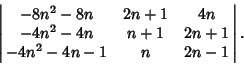(23)

Specific examples having small entries include(24)

(Guy 1989, 1994).

See also Circulant Determinant, Cofactor, Hessian Determinant, Hyperdeterminant, Immanant, Jacobian, Knot Determinant, Matrix, Minor, Permanent, Vandermonde Determinant, Wronskian

References

Arfken, G. Determinants.'' §4.1 in Mathematical Methods for Physicists, 3rd ed. Orlando, FL: Academic Press, pp. 168-176, 1985.

Guy, R. K. Unsolved Problems Come of Age.'' Amer. Math. Monthly 96, 903-909, 1989.

Guy, R. K. A Determinant of Value One.'' §F28 in Unsolved Problems in Number Theory, 2nd ed. New York: Springer-Verlag, pp. 265-266, 1994.